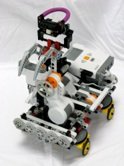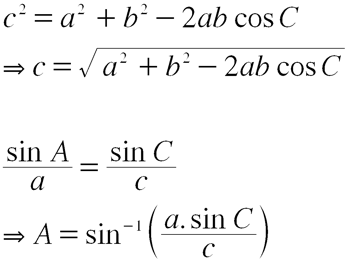Wall tracking robot

July 2008This project uses the synchronous drive chassis I developed to locate a wall and then drive alongside the wall in parallel. The idea is that the robot uses the radar to compute the angle to the wall, and then adjusts its steering to set the wheels pointing parallel to the wall. Of course the robot has to take periodic measurements to ensure that it is not deviating from the chosen course!

Theory

The basic idea is to take three radar measurements facing the wall and from the distances returned determine whether the robot is parallel to the wall, or turned to the left or right relative to the wall face. For this we’ll use some trigonometry to solve for the angle between the robot and the wall. First off, the angle we are looking for is given as the letter A in the figure below. If the robot is facing the wall straight on then A = 90 degrees.

To solve for A I used the Law of Cosines and the Law of Sines using the measurements obtained from the DISt-Nx sensor. To begin, we measure the distances from the robot face to the wall as follows:Programming

Here is the sample program that runs this algorithm:

int d1, d2, d3;

int line;

float c, angleToWall, sinA;

float fd1, fd2, fd3;

initialiseRobot();

sayHello();

// Algorithm

// Drive forward to find a wall

// Stop

// Turn radar right by 45 degrees

// Measure d1

// Turn radar left by 45 degrees

// Measure d2

// Turn radar left by 45 degrees

// Measure d3

line = 2;

// drive straight forward until we reach a wall

d1 = GetDistance();

while(d1 >= kDistToWallThreshold) {

motor[driveMotor] = kGoForwardFullSpeed;

wait10Msec(10);

d1 = GetDistance();

}

motor[driveMotor] = 0;

d1 = d2 = d3 = 0;

do {

d1 = GetDistance();

} while(d1 == 0);

do {

d2 = GetDistance();

} while(d2 == 0);

do {

d3 = GetDistance();

} while(d3 == 0);

// and reset

if( (d1 >= d2) && (d2 <= d3) ) {

nxtDisplayTextLine(line++, "Straight on");

} else

if( (d1 <= d2) && (d2 <= d3) ) {

nxtDisplayTextLine(line++, "Turned left");

} else

if( (d1 >= d2) && (d2 >= d3) ) {

nxtDisplayTextLine(line++, "Turned right");

} else {

nxtDisplayTextLine(line++, "Unknown");

}

nxtDisplayTextLine(line++, "d1 = %d", d1);

nxtDisplayTextLine(line++, "d2 = %d", d2);

nxtDisplayTextLine(line++, "d3 = %d", d3);

// now compute the angle of the robot to the wall

// Step 1: use law of Cosines to compute distance c

// c^2 = d1^2 + d2^2 - 2*d1*d2* cos 45

//

// scale back the measurements

fd1 = d1/10.0;

fd2 = d2/10.0;

fd3 = d3/10.0;

c = (fd1*fd1) + (fd2*fd2) - (2 * fd1 * fd2 * kCos45);

c = sqrt(c);

// now use the law of sines to compute the angle of the robot

// sin A = (d1 * sin 45) / c

sinA = (fd1 * kSin45) / c;

nxtDisplayTextLine(line++, "Angle=%f", angleToWall);

// turn wheels parallel to wall angle

// and off we go

motor[driveMotor] = kGoForwardFullSpeed;

wait10Msec(200);

motor[driveMotor] = 0;

while(1) {}

}

Last updated 3 September, 2008

All content © 2008 Mark Crosbie  mark@mastincrosbie.com

LEGO® is a trademark of the LEGO Group of companies which does not sponsor, authorize or endorse this site. This site, its owner and contents are in no way affiliated with or endorsed by the LEGO Group. For more please read the LEGO Fair Play policy.